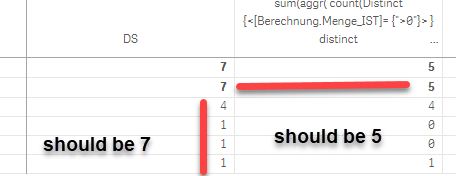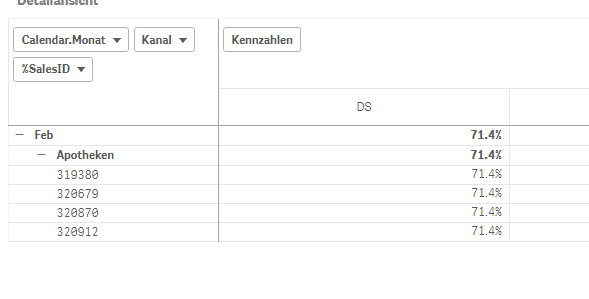Qlik Sense App Development

Discussion board where members can learn more about Qlik Sense App Development and Usage.

Announcements
QlikWorld 2020: Join us May 11 - 14, 2020 in Phoenix, AZ. Register early and save \$400. Learn More
Highlighted
New Contributor II

count- every row should have the same result (Total)

Hello

I would like, to have in every row the result from the count. Like in column DS=7.I try with SUM(TOTAL Aggr(count({<[Berechnung.Menge_IST]= {">0"}> } distinct  [Berechnung.SalesLine.LINENUM]), [%SalesID] ))/SUM(TOTAL Aggr( Count(Distinct [Berechnung.SalesLine.LINENUM]), [%SalesID] ))

but the problem is, i have the same result i every column. For every Kanal should be other result.When i select specfic Month Feb. and the "Kanal" then is the result true:How i can became in overal the right total?

Thank for you help.

1 Solution

Accepted SolutionsMVP

Re: count- every row should have the same result (Total)

May be try this

SUM(TOTAL <[Calendar.Monat]> Aggr(count({<[Berechnung.Menge_IST]= {">0"}> } distinct  [Berechnung.SalesLine.LINENUM]), [%SalesID] ))/SUM(TOTAL <[Calendar.Monat]> Aggr( Count(Distinct [Berechnung.SalesLine.LINENUM]), [%SalesID] ))